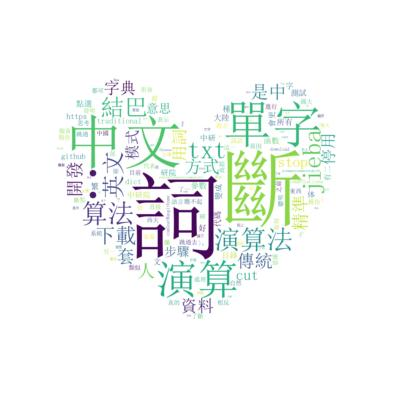# 文字雲# 安裝套件

pip install jieba wordcloud matplotlib opencv-python

# WorldCloud

from collections import Counter

import jieba
import pylab as plt
from wordcloud import WordCloud
import numpy as npimport cv2#載入停用詞
with open('stops.txt','r', encoding='utf-8') as f:
#載入文章with open("wc.txt",'r', encoding='utf-8') as f:
txt=txt.replace(" ","").replace("/","").replace("\"","")
#載入結巴中文字典
jieba.set_dictionary('dict.txt')
terms=[t for t in jieba.cut(txt, cut_all=True) if t not in stops]
#print(Counter(terms))
img=wordcloud.generate_from_frequencies(frequencies=Counter(terms))
plt.figure(figsize=(4,4))

#bilinear 雙線性插值法，讓文字不會有鋸齒狀
plt.imshow(img, interpolation="bilinear")
plt.axis("off")
plt.show()


# 遮色片